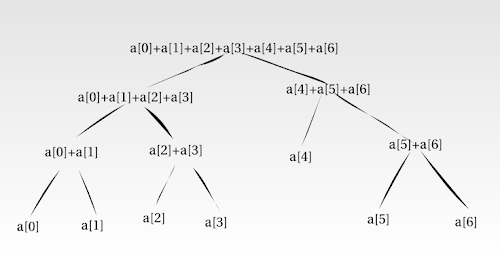## Monday, October 7, 2013

### Range trees and profiling in Haskell

Range trees. Those of my friends who've had a lot of programming competition experience would be well-acquainted with them. They're neat recursive data structures which I thought would make an excellent toy project for me to learn a bit more about performance profiling in Haskell.

### ...range trees?

A range trees is a binary-tree-based data structure used to implement range queries.

1D range query problem. Given a semigroup (i.e. an associative binary operator $\bullet$ over a domain $S$), we seek a data structure that implements the following operations over some virtual array a:

$\textrm{update}(x,v)$: set $\texttt{a}[x] := v$

$\textrm{query}(x_1,x_2)$ [given $x_1 \leq x_2$]: return $\texttt{a}[x_1] \bullet \texttt{a}[x_1+1] \bullet \cdots \bullet \texttt{a}[x_2-1]$

For example, the range minimum query problem requires a data structure that updates integers in an array and answers queries of the form What is the lowest element in the range $[x_1,x_2)$?. The range sum query problem requires a data structure answering queries like what is the sum of elements 4 to 17 inclusive?, and so on.

Range trees offer us $O(\lg W)$ updates and queries using $O(W)$, where $W$ is the size of the array. They're constructed as balanced binary trees where each leaf corresponds to an element in the array (ordered left to right, sensibly enough), and where each non-leaf node contains the sum of all the leaves underneath it.Schematic of a range tree that calculates range sums for an array a. The root node contains the sum of the entire array, its children contain the sums of the left and right halves respectively, and so on. The leaves contain the values (sums, if you will) of individual array elements.

When the value of an array element changes, only $O(\lg W)$ tree nodes need to be updated. Likewise, querying for the sum of a range of elements also only requires looking at the values stored in $O(\lg n)$ nodes. (In the example diagram above, finding the sum from a to a inclusive requires looking at three nodes: the ones storing the sums for a, a + a, and a.)

Here’s a first pass attempt at implementing a range tree in Haskell:

data RangeTree = RangeTree {width :: Int, tree :: RangeTreeNode}

newRangeTree w = RangeTree {width = w, tree = newRangeTreeNode 0 w}

update :: RangeTree -> Int -> Int -> RangeTree
update rt x v = rt {tree = updateRangeTreeNode (tree rt) 0 (width rt) x v}

query :: RangeTree -> Int -> Int -> Int
query rt = queryRangeTreeNode (tree rt) 0 (width rt)

-- Internals

data RangeTreeNode =
Leaf Int
| Branch RangeTreeNode Int RangeTreeNode

rtnValue :: RangeTreeNode -> Int
rtnValue (Leaf v) = v
rtnValue (Branch _ v _) = v

newRangeTreeNode :: Int -> Int -> RangeTreeNode
newRangeTreeNode x1 x2
| x1 == x2-1 = Leaf 0
| otherwise = Branch
(newRangeTreeNode x1 ((x1+x2) div 2))
0
(newRangeTreeNode ((x1+x2) div 2) x2)

updateRangeTreeNode :: RangeTreeNode -> Int -> Int -> Int -> Int -> RangeTreeNode
updateRangeTreeNode rtn x1 x2 x' v
| x1 > x' || x2 <= x'
= rtn
| otherwise   = case rtn of
Leaf _ -> Leaf v
Branch l _ r -> Branch l' (rtnValue l' + rtnValue r') r'
where
l' = updateRangeTreeNode l x1 ((x1+x2) div 2) x' v
r' = updateRangeTreeNode r ((x1+x2) div 2) x2 x' v

queryRangeTreeNode :: RangeTreeNode -> Int -> Int -> Int -> Int -> Int
queryRangeTreeNode rtn x1 x2 x1' x2'
| x1' >= x2 || x1 >= x2'
= 0
| x1' <= x1 && x2' >= x2
= rtnValue rtn
| otherwise
= queryRangeTreeNode l x1 ((x1+x2) div 2) x1' x2'
+ queryRangeTreeNode r ((x1+x2) div 2) x2 x1' x2'
where
Branch l _ r = rtn


The implementation is quite standard. The module exposes the functions update and query, which are wrappers for the recursive functions updateRangeTreeNode and queryRangeTreeNode.

Let's see how this runs on some random data sets: